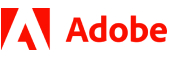New update is available. Click here to update.
Last Updated: 18 Jun, 2017

# Find Duplicate

Easy+15 more companies

## Problem statement#### You have been given an integer array/list(ARR) of size N which contains numbers from 0 to (N - 2). Each number is present at least once. That is, if N = 5, the array/list constitutes values ranging from 0 to 3 and among these, there is a single integer value that is present twice. You need to find and return that duplicate number present in the array.

##### Note :
``````Duplicate number is always present in the given array/list.
``````
##### Input format :
``````The first line contains an Integer 't' which denotes the number of test cases or queries to be run. Then the test cases follow.

First line of each test case or query contains an integer 'N' representing the size of the array/list.

Second line contains 'N' single space separated integers representing the elements in the array/list.
``````
##### Output Format :
``````For each test case, print the duplicate element in the array/list.

Output for every test case will be printed in a separate line.
``````
##### Constraints :
``````1 <= t <= 10^2
0 <= N <= 10^3
Time Limit: 1 sec
``````